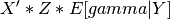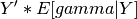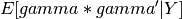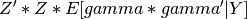# statsmodels.regression.mixed_linear_model.MixedLM.Estep¶

`MixedLM.``Estep`(fe_params, cov_re, scale)[source]

The E-step of the EM algorithm.

This is for ML (not REML), but it seems to be good enough to use for REML starting values.

Parameters: fe_params : 1d ndarray The current value of the fixed effect coefficients cov_re : 2d ndarray The current value of the covariance matrix of random effects scale : positive scalar The current value of the error variance m1x : 1d ndarray sum_groups, where X and Z are the fixed and random effects covariates, gamma is the random effects, and Y is the observed data m1y : scalar sum_groupsm2 : 2d ndarray sum_groupsm2xx : 2d ndarray sum_groups